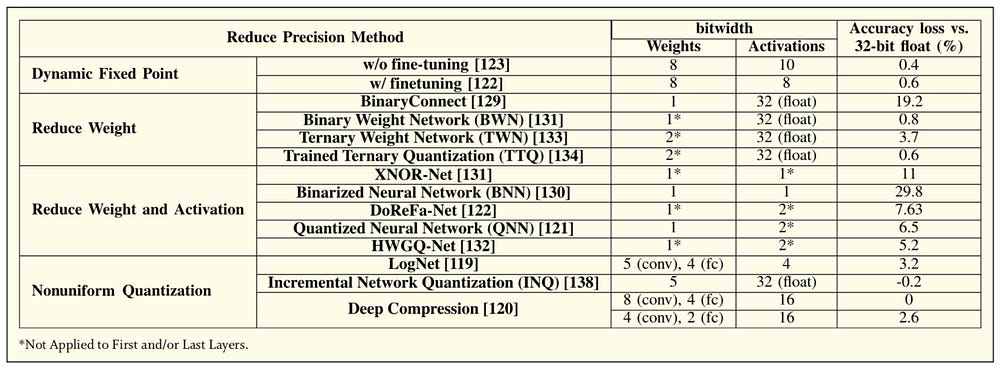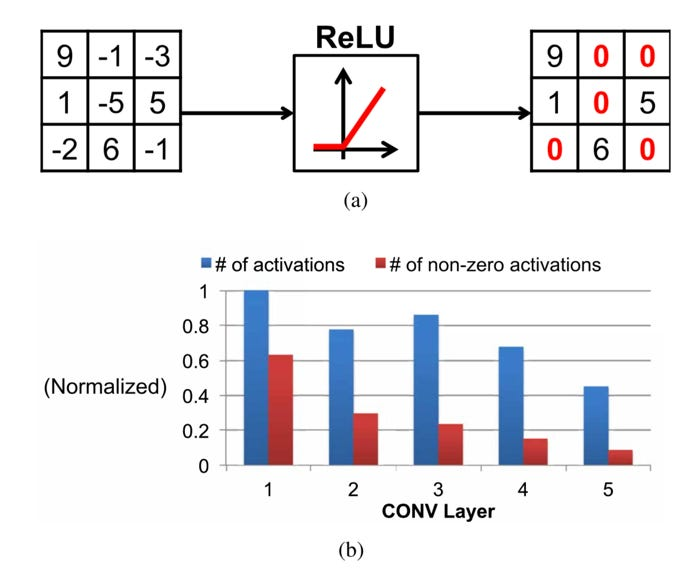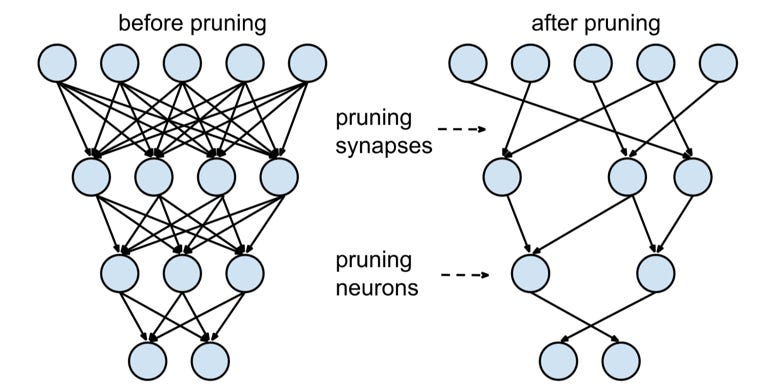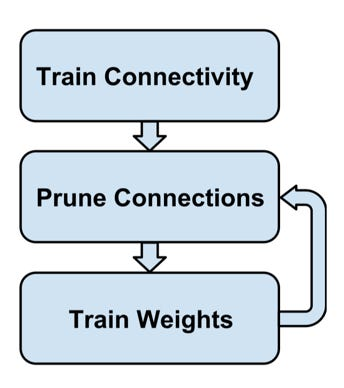# Very Efficient Deep Neural Network

Source: Deep Learning on Medium

Author: Jimmy Jin, researcher of Corpy&Co., Inc. Specialized in Medical Image Processing.

Abstract: While DNNs deliver state-of-the-art accuracy on many AI tasks, it comes at the cost of high computational complexity. In real applications, energy-efficiency algorithms play a crucial part in real-time and small-scale systems. When we start to consider this speed-up optimization, trade-offs are considered between different algorithms. The article will briefly discuss several different approaches on efficient inference. The basic idea is to maximize accuracy without much consideration of the implementation complexity.

### Reduce Precision

Quantization involves mapping data to a smaller set of quantization levels. The ultimate goal is to minimize the error between the reconstructed data from the quantization levels and the original data. The number of quantization levels reflects the precision and ultimately the number of bits required to represent the data (usually log2 of the number of levels); thus, reduced precision refers to reducing the number of levels, and thus the number of bits. The benefits of reduced precision include reduced storage cost and/or reduced computation requirements.

There are several ways to map the data to quantization levels. The simplest method is a mapping with uniform distance between each quantization level. Another approach is to use a simple mapping function such as a log function where the distance between the levels varies; this mapping can often be implemented with simple logic such as a shift.

The key techniques used in recent work to reduce precision are summarized in Table 1; both uniform and nonuniform quantizations applied to weights and activations are explored. The impact on accuracy is reported relative to a baseline precision of 32-bit floating point, which is the default precision used on platforms such as GPUs and CPUs.Table 1 Methods to Reduce Numerical Precision for AlexNet. Accuracy Measured for Top-5 Error on ImageNet

### Exploiting Activation Statistics

ReLU is a popular form of nonlinearity used in DNNs that sets all negative values to zero. As a result, the output activations of the feature maps after the ReLU are sparse. To compression, computer can choose to skip those zero-valued activations to reduce energy and cost.ReLU

### Network Pruning

To make network training easier, the networks are usually overparameterized. Therefore, a large amount of the weights in a network are redundant and can be removed (i.e., set to zero). This process is called network pruning.Network pruning

Aggressive network pruning often requires some fine-tuning of the weights to maintain the original accuracy. The low- saliency weights were removed and the remaining weights were fine-tuned; this process was repeated until the desired weight reduction and accuracy were reached.The process of network pruning

In all, great deals of work can be utilized to make deep neural network more efficient. As the basic image recognition problem has already reached the start of the art performance. More researches on more efficient deep neural network will be deployed in the future, and a crucial step for industry.

### Reference

ViVienne Sze, Efficient Processing of Deep Neural Networks: A Tutorial and Survey, IEEE, Vol. 105, №12.